# How to Use VSTACK and HSTACK in ExcelClaudia Buckley#### Join the Excel conversation on Slack

Ask a question or join the conversation for all things Excel on our Slack channel.

In March 2022, Microsoft released 14 new Excel functions specifically for working with text and dynamic arrays. With these functions, you no longer need to be an advanced Excel user to easily split text or combine, resize, and reshape arrays.

## What are VSTACK and HSTACK in Excel?

Two of those functions, VSTACK and HSTACK, help you to easily combine multiple lists into one list in Excel. Stacking, or appending, tables and lists on top of or next to each other can now be done effortlessly. This saves time because there will be less need for complex nesting and intermediate-to-advanced tools like Power Query

## Availability

Both VSTACK and HSTACK can be found within the Lookup & Reference category of the Excel Function Library. If they’re not, then it means you don’t have a current Microsoft 365 subscription. The good news is, you can get access to it for free using Excel for the Web.

Use this free exercise file to practice along with the tutorial.

## How to use the VSTACK function

VSTACK uses the dynamic array environment to combine lists and arrays vertically into a single array. It has no complicated arguments or formats. The syntax of VSTACK is:

````=VSTACK (array1, [array2], ...)`
```

Below, we can combine the A1:A4 array with the B1:B4 array with the simple formula.

````=VSTACK(A1:A4,B1:B4)`
```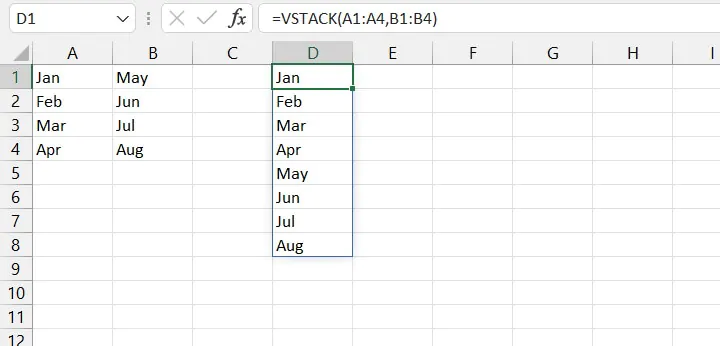As with other dynamic formulas, if there are not enough empty cells to return the result, Excel will return a #SPILL! error.

## How to use the HSTACK function

HSTACK is the horizontal version of VSTACK. It appends lists side by side so that they appear as one seamless array.

The syntax of HSTACK is:

``=HSTACK (array1, [array2], ...)``

The formula to combine the three stacks next to each other as shown below is:

````=HSTACK(A1:A5,A7:A11,A13:A17)`
```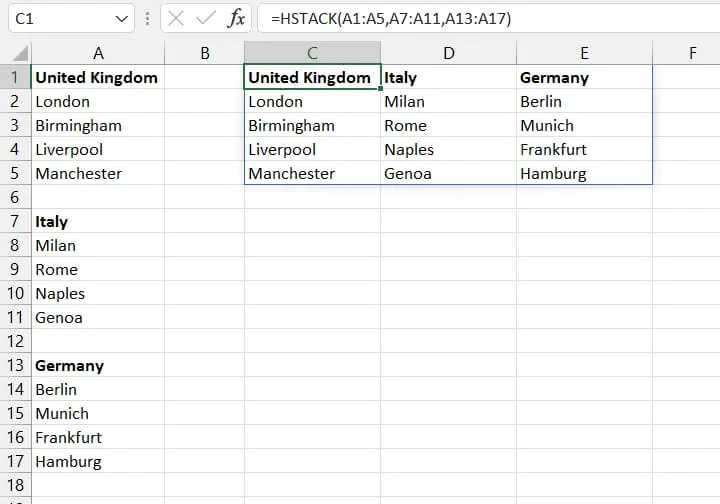## Troubleshooting

You probably won't come across any major unexpected results while working with VSTACK and HSTACK because these functions are so easy to use and understand. However, here below are a few tips that you might find handy.

### Combining ranges of different sizes

If the arrays being combined are of different dimensions, HSTACK and VSTACK will create a two-dimensional array that corresponds to the largest array being stacked. The extra cells from the smaller array will be padded with a value of #N/A.

In the following example, a 4x1 array is vertically stacked with a 4x2 array. The result is an 8x2 matrix, with the non-existent second column from the 4x1 array being filled in with #N/A values.

``=VSTACK(A1:A4,D1:E4)``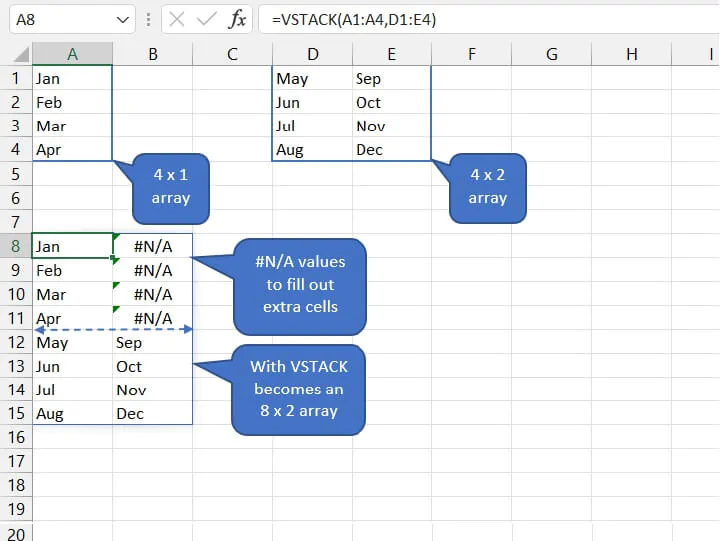The IFERROR formula can be used to “pad” the error cells with an alternate value, as shown below.

``=IFERROR(VSTACK(A1:A4,D1:E4),"")``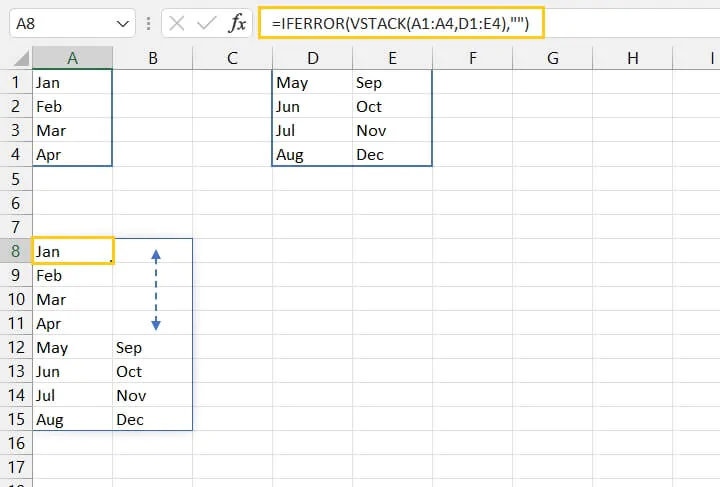The pair of empty double quotes tells Excel to return an empty cell if the VSTACK formula results in an error.

### Handling empty cells within an array

A slightly different outcome is given if the array to be stacked contains actual empty cells. In that case, those cells will display zeros.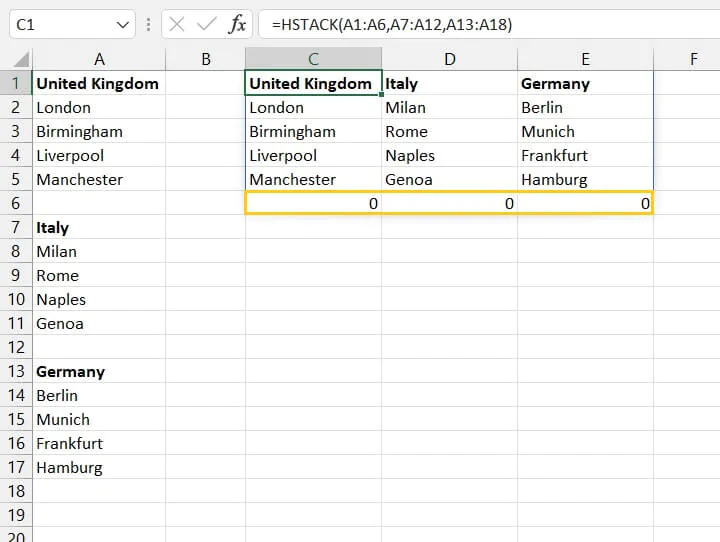A simple solution for this problem is to add a pair of empty double quotes to the end of the formula using the ampersand operator (&).

``=HSTACK(A1:A6,A7:A12,A13:A18)&""``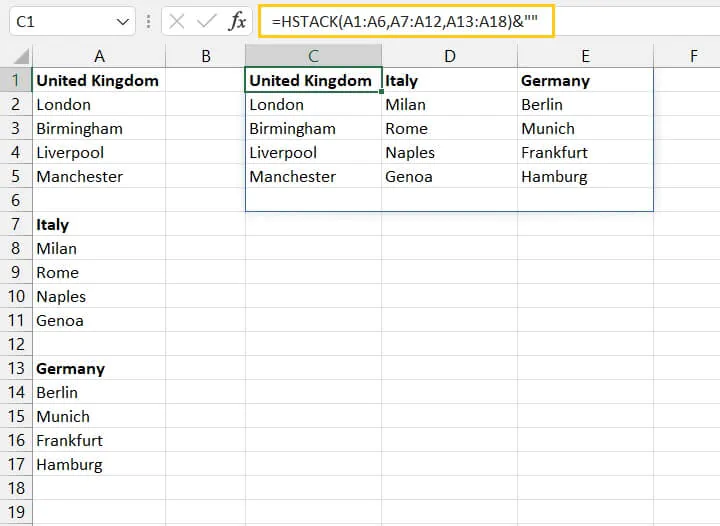## Raise your efficiency levels with Excel

These functions are a welcome solution to copy-pasting or more advanced data transformation techniques. Anyone can now append tables, lists, and arrays in Excel without being an expert.

Stay on the cutting edge of data representation by getting certified in our Basic & Advanced Excel course today. Or start from scratch with our FREE beginner-friendly Excel in an Hour course!

Ready to become a certified Excel ninja?